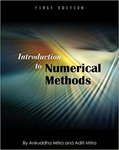## Mathematical Sciences Faculty Bookshelf#### Title

Introduction to Numerical Methods

#### Description

Georgia Southern University faculty members Aditi Mitri and Aniruddha Mitra co-authored Introduction to Numerical Methods.

Book Summary: Written for sophomore-level students in mechanical engineering programs and designed to give them the math preparation they need to succeed in higher level mechanical engineering courses, Introduction to Numerical Methods incorporates theory and worked-out engineering-related problems that apply that theory, as well as relevant laboratory exercises.

Ideally suited to one-semester, three-credit, problem solving session-based courses, the book covers errors in computation, rounding and chopping, solving equations with numerical techniques, matrixes and vectors, and complex numbers. The material also includes an introduction to optimization, linear programming problems, and instruction in probability and statistics.

It should be noted that many of the exercises in the book suggest the use of a Ti-83 calculator, and that tips for using this calculator successfully are integrated into the text. Introduction to Numerical Methods is a well-organized, useful addition to undergraduate course work in engineering programs, especially in the mechanical discipline.

7-29-2016

#### Publisher